# NCERT Solutions for Class 12 Physics Chapter 12 Atoms

In this chapter, we provide NCERT Solutions for Class 12 Physics Chapter 12 Atoms for English medium students, Which will very helpful for every student in their exams. Students can download the latest NCERT Solutions for Class 12 Physics Chapter 12 Atoms pdf, free NCERT solutions for Class 12 Physics Chapter 12 Atoms book pdf download. Now you will get step by step solution to each question.

## NCERT Solutions for Class 12 Physics Chapter 12 Atoms

Question 1.
Choose the correct alternative from clues given at end of the each statement:
(a)
The size of the atom in Thomson’s model is the atomic size in Rutherford’s model.(much greater than/no different from/much less than.)
(b) In the ground state of…………… electrons are in stable equilibrium, while in ……………..  electrons always experience a net force. (Thomson’s model/Rutherford’s model.)
(c) A classical atom based on…………. is doomed to collapse.
(Thomson’s model/Rutherford’s model.)
(d) An atom has a nearly continuous mass distribution in a………….. but has a highly non­uniform mass distribution in  (Thomson’s model/Rutherford’s model.)
(e) The positively charged part of the atom possesses most of the mass in………….
(Rutherford’s model/both the models.)
(a) no different from
(b) Thomson’s model; Rutherford’s model.
(c) Rutherford’s model.
(d) Thomson’s model, Rutherford’s model.
(e) both the models.

Question 2.
Suppose you are given a chance to repeat the alpha- particle scattering experiment using a thin sheet of solid hydrogen in place of the gold foil. (Hydrogen is a solid at temperature 14 K). What results do you expect ?
α-particle is much heavier than hydrogen nuclei. Therefore, α-particles pass through solid hydrogen without deflection. In other words, α-particles are not scattered by solid hydrogen.

Question 3.
What is the shortest wavelength present in the Paschen series of spectral lines ?
The wavelength of the spectral lines forming Paschen series is given by

Question 4.

A difference of 2.3 eV separates two energy levels in an atom. What is the frequency of radiation emitted when the atom transits from the upper level to the lower level ?Question 5.
The ground state energy of hydrogen atom is -13.6 eV. What are the kinetic and potential energies of the electron in this state ?
K.E. = -E (Total energy)
= -(-13.6) = 13.6 eV
P.E. = 2 X E = 2 X (-13.6)
= -27.2 eV
A hydrogen atom initially in the ground level absorbs a photon which excites it to the
n = 4 level. Determine the wavelength and frequency of photon.
We know, energy of an electron in the nth orbit of hydrogen atom is given by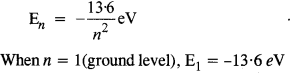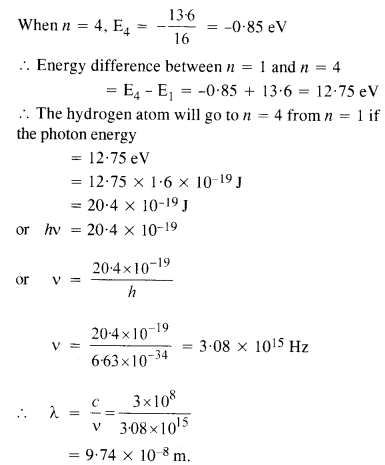Question 7.
Using the Bohr’s model, calculate the speed of the electron in a hydrogen atom in the n = 1,2 and 3 levels. (b) Calculate the orbital period in each of these levels.
(a) Speed of an electron in nth orbit of hydrogen atom is given by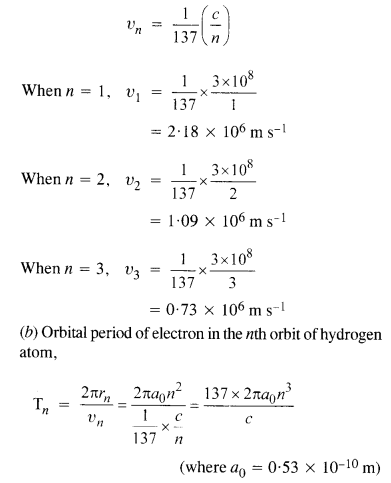Question 8.
The radius of the innermost electron orbit of a hydrogen atom is 5.3 x I0-11 m. What are the radii of the n = 2 and n = 3 orbits?
We know, radius of nth orbit of hydrogen atom is given by
rn = r0n2, where r0 = 5.3 x 10-u m is the radius of
the innermost orbit of hydrogen atom.
When n = 2, r2 = 5.3 x 10-u X 4
= 2.12 X 10-10m
When n = 3,
= 5.3 x 10-11 x 9
= 4.77 x 10-10m.

Question 9.
A 12.75 eV electron beam is used to bombard gaseous hydrogen at room temperature. What series of wavelengths will be emitted ?

Question 10.
In accordance with the Bohr’s model, And the quantum number that characterises the earth’s revolution around the sun in an orbit of radius 1.5 x 1011 m with orbital speed
3 x 1014 m s-1. (Mass of earth = 6.0 x 1024 kg.)
According to Bohr’s postulate of quantization of angular momentumQuestion 11.
Answer the following questions, which help you understand the difference between Thomson’s model and Rutherford’s model better.
(a) Is the average angle of deflection of a-particles by a thin gold foil predicted by Thomson’s model much less, about the same, or much greater than that predicted by Rutherford’s model ?
(b) Is the probability of backward scattering (i.e., scattering of a-particles at angles greater than 90°) predicted by Thomson’s model much less, about the same, or much greater than that predicted by Rutherford’s model ?
(c) Keeping other factors fixed, it is found experimentally that for small thickness t, the number of a-particles scattered at moderate angles is proportional to What clue does this linear independence on t provide ?
(d) In which model is it completely wrong to ignore multiple scattering for the calculation of average angle of scattering of a-particles by a thin foil ?
Ans:
(a) It is about the same because we are considering average deflection angle.
(b) It is much less than the predicted value because the massive core is absent in Thomson’s model.
(c) It suggests that the scattering of a-particles is primarily because of the single collision.
(d) In Thomson’s model of atom. Multiple collisions are required to be considered in this model because positive charge is spread throughout in this model.

Question 12.
The gravitational attraction between electron and proton in a hydrogen atom is weaker than the coulomb attraction by a factor of about 10-40. An alternative way of looking at this fact is to estimate the radius of the first Bohr orbit of a hydrogen atom if the electron and proton were bound by gravitational attraction. You will find the answer interesting.
If electron and proton were bound by gravitational attraction, thenIt is astonishing this value of r is much greater than the size of universe.

Question 13.
Obtain an expression for the frequency of radiation emitted when a hydrogen atom
de-excites from level it to level (n – 1). For large it, show that this frequency equals the classical frequency of revolution of the electron in the orbit.
The energy of an electron in the nth orbit of hydrogen atom is given by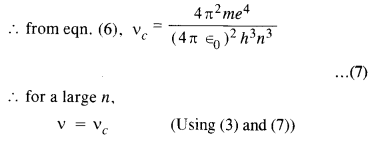i.e. frequencies are equal. This is called the Bohr’s correspondence principle.

Question 14.
Classically, an electron can be in any orbit around the nucleus of an atom. Then what determines the typical atomic size ? Why is an atom not, say, thousand times bigger than its typical size ? The question had greatly puzzled Bohr before he arrived at his famous model of the atom that you have learnt in the text. To stimulate what he might well have done before his discovery, let us play as follows with the basic constants of nature and see if we can get a quantity with the dimensions of length that is roughly equal to the known size of an atom (~ 10-10m).
(a) Construct a quantity with the dimensions of length from the fundamental constants e, me, and c. Determine its numerical value.
(b) You will find that the length obtained in («) many orders of magnitude smaller than the atomic dimensions. Further, it involves c. But energies of atoms are mostly in non-relativistic domain where c is not expected to play any role. This is what may have suggested Bohr to discard c and look for ‘something else’ to get the right atomic size. Now, the Planck’s constant h had already made its appearance elsewhere. Bohr’s great insight lay in recognising that h, me, and e will yield the right atomic size. Construct a quantity with the dimension of length from h, mg, and e and confirm that its numerical value has indeed the correct order of magnitude.
(a) Here, the dimensional formula of e is A1T1, the dimensional formula of me is M1, dimensional formula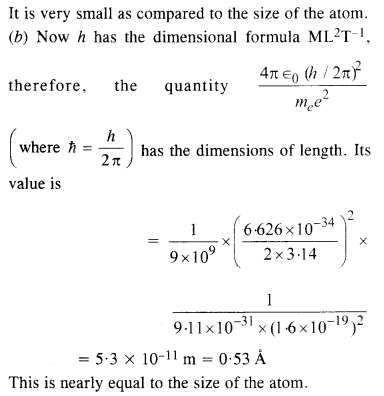Question 15.
The total energy of an electron in the first excited state of the hydrogen atom is about
– 3.4 eV.
(a) What is the kinetic energy of the electron in this state ?
(b) What is the potential energy of the electron in this state ?
(c) Which of the answers above would change if the choice of the zero of potential energy is changed ?
(a) K.E. = -E = – (-3.4 eV) = 3.4 eV
E. = 2E = 2 x (-3.4 eV)
= -6.8 eV
(b) Kinetic energy does not depend upon the choice of zero of potential energy. Therefore, its value remains unchanged. However, the potential energy gets changed with the change in the zero level of potential energy.

Question 16.
If Bohr’s quantisation postulate (angular momentum = nh/2π) is a basic law of nature, it should be equally valid for the case of planetary motion also. Why then do we never speak of quantisation of orbits of planets around the sun ?
Applying the Bohr’s quantization postulate,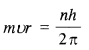i.e., n is very large. Since n is very large, the difference between the two successive energy or angular momentum levels is very small and the levels may be considered continuous.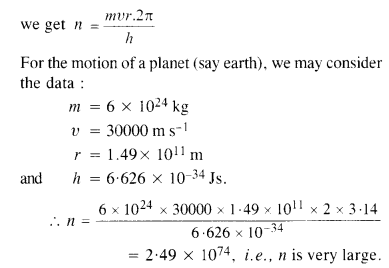Question 17.
Obtain the first Bohr’s radius and the ground state energy of a ‘muonic hydrogen atom’ (i.e. an atom in which a negatively charged muon (μ-1) of mass about 207 me orbits around a proton).
Here mass of the particle revolving around the proton isAll Chapter NCERT Solutions For Class 12 Physics

—————————————————————————–

All Subject NCERT Solutions For Class 12

*************************************************

I think you got complete solutions for this chapter. If You have any queries regarding this chapter, please comment on the below section our subject teacher will answer you. We tried our best to give complete solutions so you got good marks in your exam.

If these solutions have helped you, you can also share ncertsolutionsfor.com to your friends.